# 10 minutes to learn how to play excel easily with Python

2022-02-02 00:46:27

### Preface

When you need to be right every day `Excel` Do a lot of repetitive operations , If you only rely on manual work, it will waste time , And very boring , Fortunately `Python` It provides us with many operations `Excel` Module , It can free us from tedious work .

Today I will share with you a quick processing `Excel` Module `openpyxl`, Its functions are more complete than other modules , Enough to deal with daily problems .

### openpyxl install

Enter... Directly at the command prompt .

``````pip install openpyxl
Copy code ``````

Or use watercress image to install .

``````pip install -i https://pypi.douban.com/simple openpyxl
Copy code ``````

After successful installation , Let's see how to use

### open / Create Workbook

Sample Workbook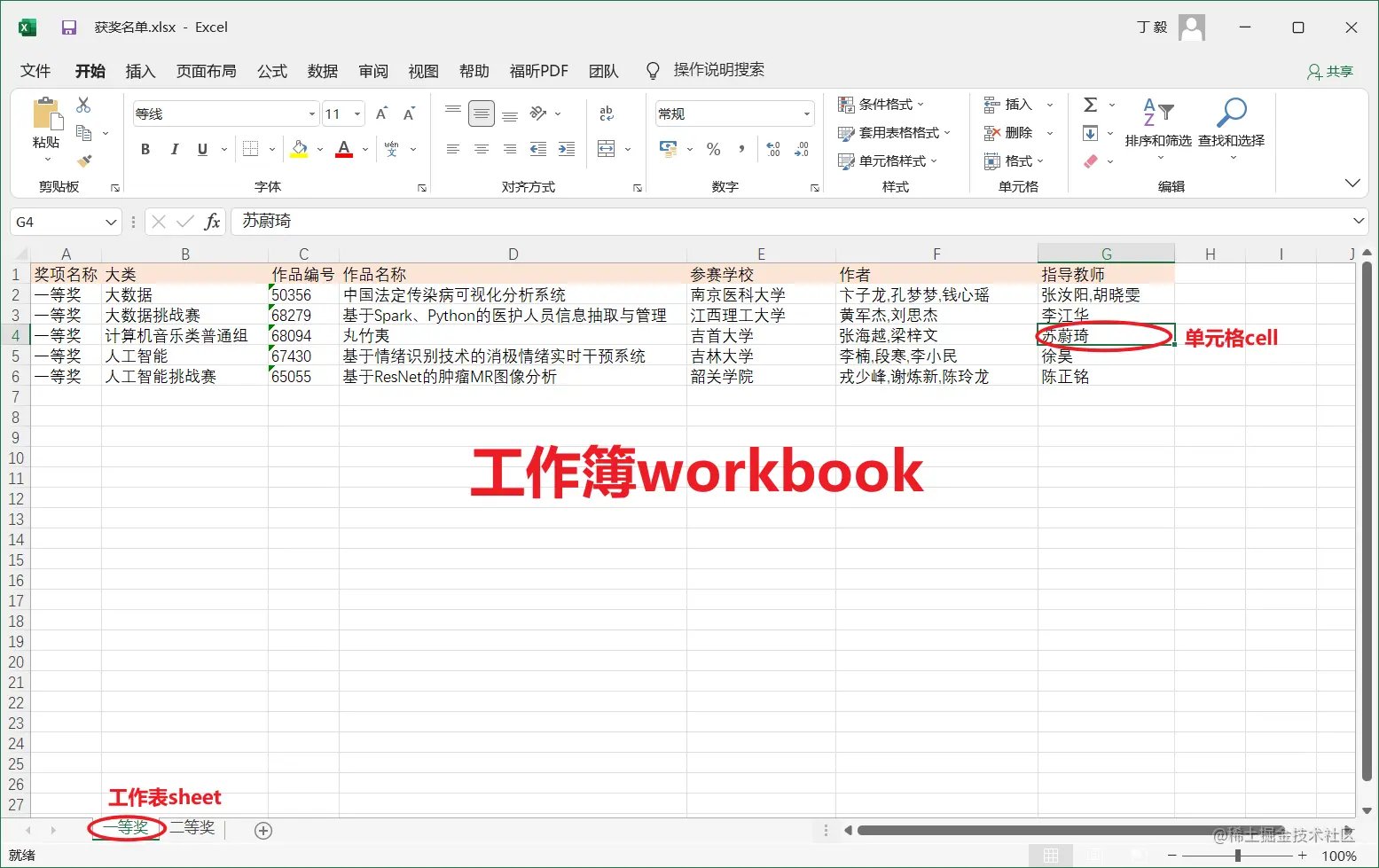Worksheet 【 The first prize 】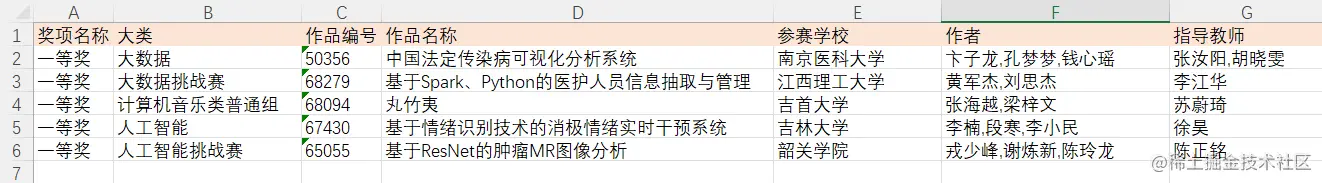Worksheet 【 The second prize 】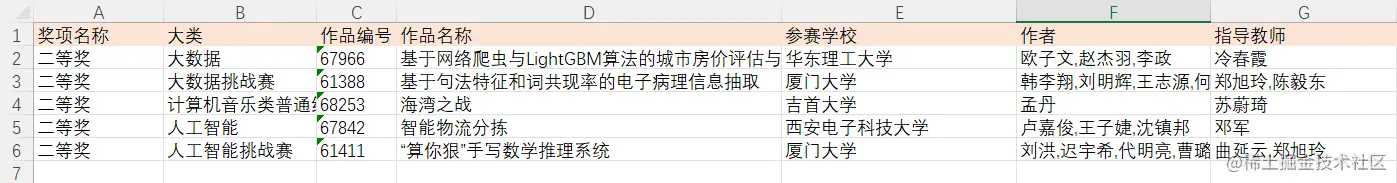(1) Open local workbook

``````>>> from openpyxl import load_workbook
>>> wb = load_workbook(' Winning list .xlsx')
Copy code ``````

(2) Create an empty workbook

``````>>> from openpyxl import Workbook
>>> wb1 = Workbook()
Copy code ``````

### Access worksheet

Create a new worksheet , The insertion position can be specified （`0: First place ,-1: At the end of `）.

``````>>> wb.create_sheet('new_sheet', 0)
<Worksheet "new_sheet">
Copy code ``````

Get all worksheets in the workbook .

``````>>> wb.sheetnames
['new_sheet', ' The first prize ', ' The second prize ']
Copy code ``````

Use list derivation traversal to get all worksheet names .

``````>>> [sheet.title for sheet in wb]
['new_sheet', ' The first prize ', ' The second prize ']
Copy code ``````

Use `wb[sheetname]` To get a worksheet object

``````>>> wb[' The second prize ']
<Worksheet " The second prize ">
Copy code ``````

Get activity table ( That is, the worksheet that appears first when you open the workbook ).

``````>>> wb.active
<Worksheet " The first prize ">
Copy code ``````

Get worksheet row and column information .

``````>>> sheet1 = wb[' The first prize ']
>>> sheet1.max_column
7
>>> sheet1.max_row
6
Copy code ``````

### Get cell information

#### Access a cell

``````>>> sheet1['D3']
<Cell ' The first prize '.D3>
>>> sheet1.cell(row=3, column=4)
<Cell ' The first prize '.D3>
Copy code ``````

If the access unit format is added `value` Parameter will modify the value of the current cell .

``````>>> sheet1.cell(3, 4).value
' be based on Spark、Python Information extraction and management of medical staff '
>>> sheet1.cell(3, 4, value='Python')
<Cell ' The first prize '.D3>
>>> sheet1.cell(3, 4).value
'Python'
Copy code ``````

Get cell value 、 coordinate 、 Row index 、 Column index .

``````>>> sheet1['D3'].value
'Python'
>>> sheet1['D3'].coordinate
'D3'
>>> sheet1['D3'].row
3
>>> sheet1['D3'].column
4
Copy code ``````

#### Access multiple cells

Use slices to access multiple cells , The slice here is different from the list slice , List slice is Before closed after opening ,Excel The slice in is Front closed and rear closed .

(1) selection `A1:B2` Cells in the range .

``````>>> sheet1['A1':'B2']
((<Cell ' The first prize '.A1>,
<Cell ' The first prize '.B1>),
(<Cell ' The first prize '.A2>,
<Cell ' The first prize '.B2>))
Copy code ``````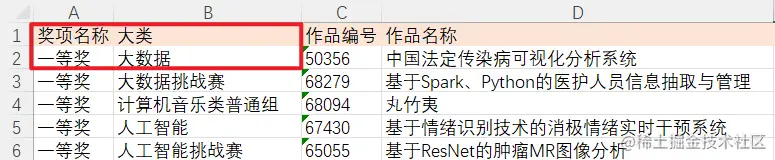Select a single column of data .

``````>>> sheet1['D']
(<Cell ' The first prize '.D1>,
...
<Cell ' The first prize '.D6>)
Copy code ``````

selection B,C Column data .

`````` >>> sheet1['B:C']
((<Cell ' The first prize '.B1>,
...
<Cell ' The first prize '.B6>),
(<Cell ' The first prize '.C1>,
...
<Cell ' The first prize '.C6>))
Copy code ``````

Select single line data .

``````>>> sheet1
(<Cell ' The first prize '.A3>,
<Cell ' The first prize '.B3>,
...
<Cell ' The first prize '.F3>,
<Cell ' The first prize '.G3>)
Copy code ``````

Select the first 2,3 Row data .

``````>>> sheet1[2:3]
((<Cell ' The first prize '.A2>,
...
<Cell ' The first prize '.G2>),
(<Cell ' The first prize '.A3>,
...
<Cell ' The first prize '.G3>))
Copy code ``````

#### Traverse to get data

Traverse the specified range by line （`B2:C3`） data .

``````>>> for row in  sheet1.iter_rows(min_row=2, max_row=3, min_col=2, max_col=3):
for cell in  row:
print(cell.coordinate)

B2
C2
B3
C3
Copy code ``````

Traverse the specified range by column （`B2:C3`） data .

``````>>> for col in  sheet1.iter_cols(min_row=2, max_row=3, min_col=2, max_col=3):
for cell in col:
print(cell.coordinate)

B2
B3
C2
C3
Copy code ``````

If `iter_rows()/iter_cols()` Specify parameters in `values_only=True`, Then only the value of the cell will be returned

Traverse all data by row .

``````>>> tuple(sheet1.rows)
((<Cell ' The first prize '.A1>,
...
<Cell ' The first prize '.G1>),
...
...
(<Cell ' The first prize '.A6>,
...
<Cell ' The first prize '.G6>))
Copy code ``````

Traverse all data by column .

``````>>> tuple(sheet1.columns)
((<Cell ' The first prize '.A1>,
...
<Cell ' The first prize '.A6>),
...
...
(<Cell ' The first prize '.G1>,
...
<Cell ' The first prize '.G6>))
Copy code ``````

### Modify worksheet

#### Cell assignment

Add a new column to calculate ` author ` The number of .

``````>>> for row_index in range(2, sheet1.max_row + 1):
sheet1.cell(row_index, 8).value = len(sheet1.cell(row_index, 6).value.split(','))
Copy code ``````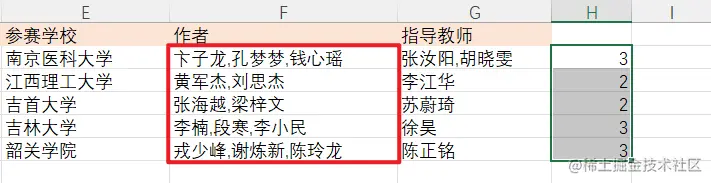Assign a value to a cell using a formula ,`H7` Count the total number of authors .

``````>>> sheet1['H7'] = '=SUM(H1:H6)'
Copy code ``````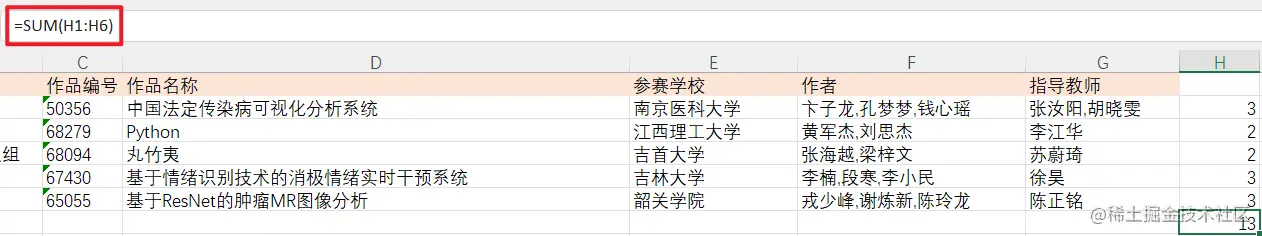#### Append a row of data

Use the list to pass in values in order .

``````>>> sheet1.append([str(n) for n in range(6)])
Copy code ``````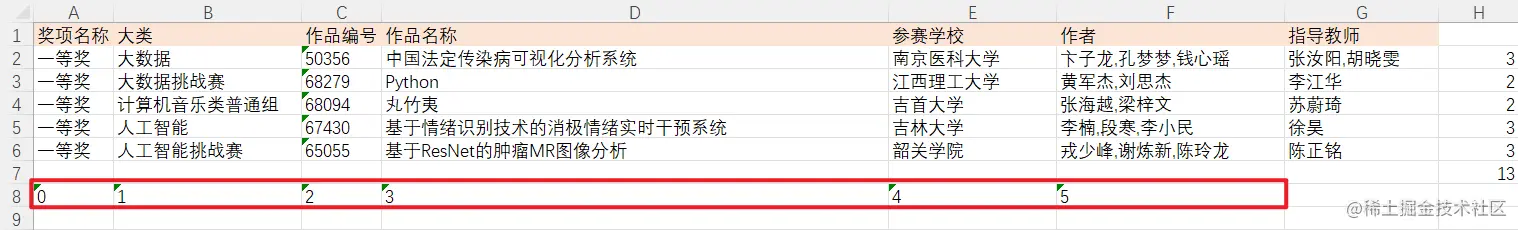Use the dictionary to specify ` Column index : The column value ` .

``````>>> sheet1.append({'A':'1','C':'3'})
Copy code ``````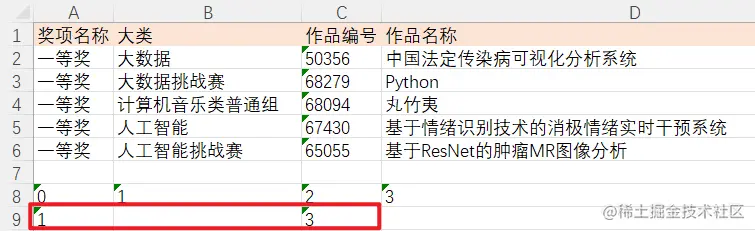#### Insert blank line

Insert a blank line at the specified position , `idx` Row index , Insertion position ;`amount` Insert the number of blank lines

``````>>> sheet1.insert_rows(idx=2, amount=2)
Copy code ``````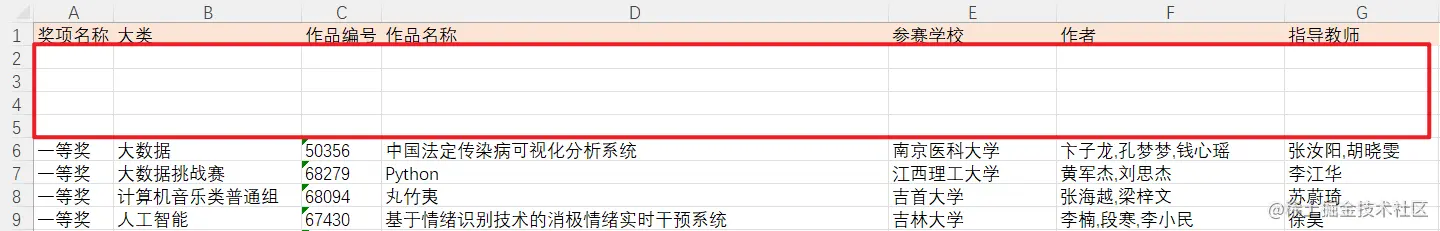#### Delete sheet

``````>>> wb.remove(wb['new_sheet'])
Copy code ``````

#### Save workbook

``````>>> wb.save(' Winning list V1.xlsx')
Copy code ``````

### Modify the style

#### typeface

Set up `B2` The cell font format is , Colors can be in hexadecimal color codes .

``````>>> from openpyxl.styles import Font

>>> new_font = Font(name=' Microsoft YaHei ', size=20, color='3333CC', bold=True)
>>> sheet1['B2'].font = new_font
Copy code ``````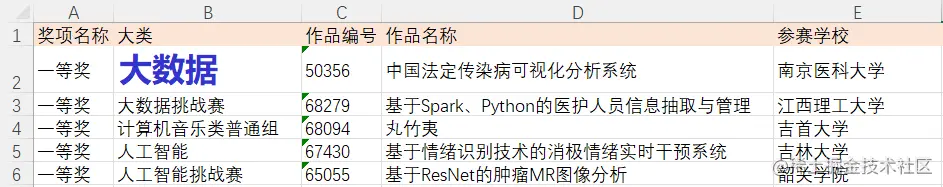#### Cell background color

``````>>> from openpyxl.styles import PatternFill, colors
>>> sheet1["A2"].fill = PatternFill("solid", fgColor=colors.BLUE)
>>> sheet1["A3"].fill = PatternFill("solid", fgColor='FF66CC')
Copy code ``````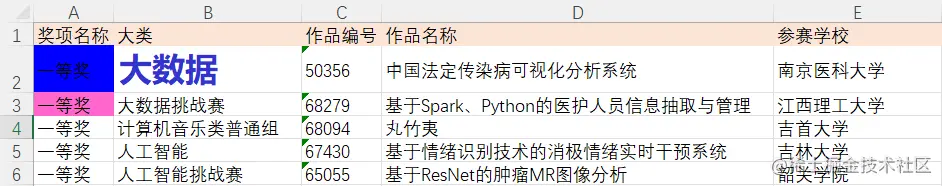#### Alignment mode

Set up `D2` Data in ` Vertical center ` and ` Horizontal center ` .

``````>>> from openpyxl.styles import Alignment
>>> sheet1['D2'].alignment = Alignment(horizontal='center', vertical='center')
Copy code ``````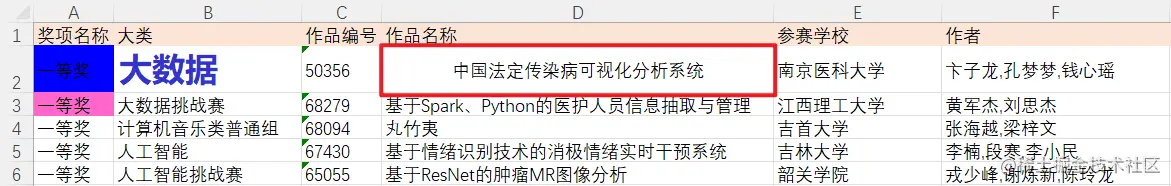#### Row height / Column width

Set the first 2 Line height 40,C Column width is 20.

``````>>> sheet1.row_dimensions.height = 40
>>> sheet1.column_dimensions['C'].width = 20
Copy code ``````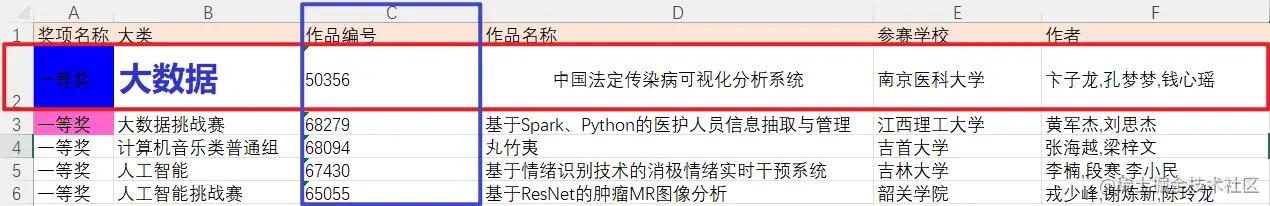#### Merge / Split cells

To merge cells, you only need to specify the cell coordinates in the upper left corner and the lower right corner .

``````>>> sheet.merge_cells('A1:C3')
Copy code ``````After splitting cells , The value of the merge range is assigned to the upper left cell A1.

``````>>> sheet.unmerge_cells('A1:C3')
Copy code ``````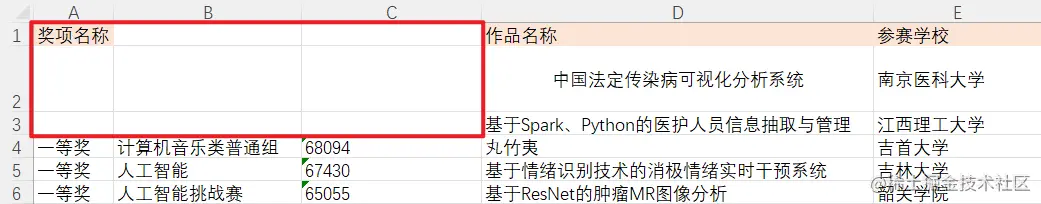For beginners `Python` Or want to get started `Python` Little buddy , You can go through the bottom Contact the author with a small card , Exchange and study together , They all come from novices , Sometimes a simple question card takes a long time , But maybe someone else's advice will suddenly realize , I sincerely hope you can make progress together .